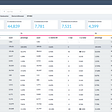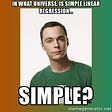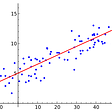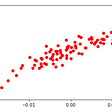# Linear Regression

1. Introduction:

Linear Regression is a Supervised Machine learning model (where training data with the label is provided) which is used for Regression tasks (to predict the values within a continuous range. e.g. sales, price).

The aim of Linear Regression is to find a line (in 2-d)/plane (in 3-d)/hyperplane(in n-d) that best fits the data.

Linear Regression could be of 2 types:

1. Simple Linear Regression: there is only one independent variable for the model to find the relationship.
2. Multiple Linear Regression: there are more than one independent variables for the model to find the relationship.

The linear Regression algorithm tries to learn the correct value for intercept and slope. At the end of the training, the model will approximate the line/plane/hyperplane that best fits the data.

2. Steps in Linear Regression: below are the steps to follow in Linear Regression:

1. Fitting a line(in 2-d)/plane (in 3-d)/hyperplane(in n-d) to the data using the Least Square method
2. Calculate R²
3. Calculate p-value for R²

Let's discuss these steps one by one to understand how the Linear Regression model actually works. Here I am taking a simple example of 2-d data and trying to find an optimal line that best fits the data.

3. Fitting a line to data using the least square method:

How to determine what line fits the data best? We try with a basic line which is always predicting the mean value as output and also try multiple rotations of the mean line, each time we check how far the line is from data points.

Below are the details 3 steps of least square or fitting the line to the data:

1. Start with a line and calculate the sum of squared residuals, which is the sum of the square of the distance of each data point from the line:

2. Now try a few more rotations of the line and calculate the sum of squared residuals.

3. Plot a graph between the sum of squared residuals vs different rotations of the line. Take the derivative of this function, the derivative tells us the slope of the function at every point. Optimal rotation is the one where slope=0. Thus, the technique is called the least square method, where we select the optimal line that gives the least sum of square residuals or least error.

At the end of the least square method technique, we will get an optimal line that best fits the data. We can superimpose the data on the selected line.

Now the next step is to determine, how good is the guess we made using the best fit line. There are several matrices to evaluate the performance of the Regression model such as , Adjusted R², Mean Squared Error (MSE), Root Mean Squared Error (RMSE) but here in this blog we will use R².

R² is also known as the coefficient of determination, which basically quantifies how much better a line fits the data.

4. Calculate R²:

R² is the ratio of variation explained by the model to the total variance.

R² value is between 0-1:

• 0 represents a model that does not explain any of the variations in the response variable around its mean. The mean of the dependent variable predicts the dependent variable as well as the regression model.
• 1 represents a model that explains all the variations in the response variable around its mean.

R² value can also be negative in some cases, R² compares the fit of the chosen model with that of a horizontal (or mean) straight line (the null hypothesis). If the chosen model fits worse than a horizontal line, then R² is negative. Note that R² is not always the square of anything, so it can have a negative value without violating any rules of math. R² is negative only when the chosen model does not follow the trend of the data, so fits worse than a horizontal line.

In the below figure, let L2 is the regression line we got using the least square method, we can see that L2 fits data better than the mean, but how to quantify that using R²?

Let variance (mean) =32, variance (L2) = 6

R² = variance(L1) — variance(L2) / variance(L1) = 32 — 6/32 = 0.81

There is 81% less variation around the L2 than mean, or the relationship between two variables (X, y) accounts for 81% of the variation.

Now, once we have R² calculated for our regression line, we need a way to determine if the R² value is statistically significant or not. What does statistically significant mean?

• Statistical significance is a determination made by an analyst that the results in the data are not explainable by chance alone.
• Statistical hypothesis testing is the method by which the analyst makes this determination.
• This test provides a p-value, which is the probability of observing results as extreme as those in the data, assuming the results are truly due to chance alone.
• A p-value of 5% or lower is often considered to be statistically significant.

Let’s see how to calculate the p-value for R². The null hypothesis in the case when determining p-value for R² would be that the selected line from the least squared method best fits the data (and obviously better than the mean line).

5. Calculate p-value for R²:

P-value is not probability value, but it is obtained by adding up probabilities. We can calculate the p-value for discrete and for continuous values as well. To calculate the p-value of discrete values we add up 3 probabilities:

1. The probability that random chance would result in the observation
2. The probability of observing something else that is equally rare
3. The probability of observing something rarer or more extreme

Example: In an experiment of tossing a coin 2 times, sample space would be {HH, HT, TH, TT}, where H means heads and T means tails. Here, we assume that the coin is fair (which is our Null hypothesis H₀). The reason we calculate the p-value is to test our null hypothesis:

p-value for getting 2 heads would be:

p-value (2 heads) = probability(random chance would result in the observation like HH) + probability(observing something else that is equally rare like TT)+ probability(observing something rarer or more extreme)

p-value (2 heads) = p (HH) + p(TT equally rare as HH) + p(extreme rare observation than HH or TT) = 0.25 + 0.25 + 0 = 0.5

Here, the probability of getting 2 heads (0.25) and the p-value of getting 2 heads (0.5) is different. The p-value of getting 2 heads is greater than the threshold (0.05) so we fail to reject the Null Hypothesis and our coin is fair, not biased even after getting 2 Heads in a row.

Calculating p-value in the case of discrete values is easy because we can list all possible outcomes, but what about the case of continuous values, if we want to measure the p-value of how tall or short people are? In practice, we use statistical distribution in the case of continuous values, for example- Normal Distribution. Below is the distribution of height measurement of Indian women (of age 15–49 years) in 1990. The whole area under the below curve indicates the probability that a person’s height is within the range of possible values.

To calculate the p-value of the distribution, we add up the percentage of area under the curve. Suppose, we want to calculate the p-value of someone who is 142 cm tall and our Null hypothesis (H₀) is that the same distribution (with an average of 155.5 cm) explains the height of 142 cm. We want to check if the 142 cm height is so far from the mean of the distribution that we reject the idea that it came from that distribution. For this, we calculate the p-value of someone who is 142 cm tall and if it is less than the threshold we can reject the Null hypothesis which means, there is some other distribution that explains the 142 cm tall height in a better way.

Also, when we are working with a distribution, we are interested in adding more extreme value to the p-value rather than the rarer value.

p-value of someone who is 142 cm tall = 2.5% area of people ≤ 142 cm of height are extreme rare + 2.5% area of the people ≥ 169 cm height are also considered as extreme rare

= 2.5% + 2.5% = 5% = 0.05

The p-value of someone who is 142 cm tall is 0.05 which is equal to the threshold value, so we can’t decide to reject or fail to reject Null Hypothesis.

Let’s calculate p-value of someone who is 141 cm tall is: 0.016 + 0.016 = 0.032 < 0.05

We can say that since the p-value of people with height 141 cm is smaller than the threshold, we reject the null hypothesis that it is explained by the original distribution, and It's special (not normal case) to measure someone with that small height and that suggests that a different distribution of heights make more sense. For example, the below green distribution makes more sense for height 141 cm:

6. End Notes:

I have covered most of the concepts behind the Linear Regression model in this blog. If you wish to know about more the mathematics behind the model and see a practical implementation using python on the Boston House Pricing Dataset, check my blog.

7. References:

--

--

--

## More from Heena Sharma

Love podcasts or audiobooks? Learn on the go with our new app.

## Best Analytics Jobs## Democratizing Experimentation Data for Product Innovations## Behavioral Analytics and what Lies ahead## Setup a Data Science Environment on your Personal Computer## Analytics services. Server-side analytics## Best 11 Data Science Programming Languages in 2020## Google News and Leo Tolstoy: Visualizing Word2Vec Word Embeddings with t-SNE## Big Data Analytics## Linear Regression## Simple Linear Regression Simplified## Linear regression## Linear Regression Assumptions